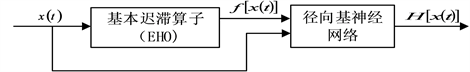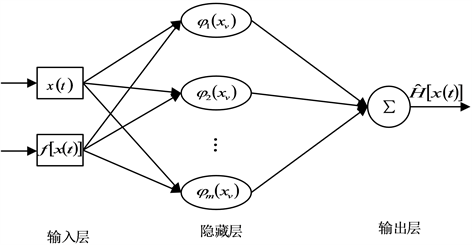﻿ 一类微驱动器的复合控制及稳定性分析

# 一类微驱动器的复合控制及稳定性分析Stability Analysis for a Class of Micro Actuator under the Compound Control

Abstract: The difference equation model of micro actuator is considered in this paper. An equivalent state space model is derived from the dynamic equation of micro actuator. According to the model, a new compound control method, including feed-forward control, PID control and radial basis network control, is proposed. The stability of the new control method is proved through constructing ac-ceptable Lyapunov function.

1. 引言

2. LuGre摩擦模型的问题描述

LuGre摩擦模型    将两个不规则的接触面想象成弹性刚毛的接触，当施加切向力时，刚毛就会像弹簧一样变形，刚毛所产生的阻力便是摩擦力。而刚毛的平均形变定义为

$\stackrel{˙}{h}=\stackrel{˙}{x}-\frac{|\stackrel{˙}{x}|}{g\left(\stackrel{˙}{x}\right)}h$ (1)

${F}_{h}={\sigma }_{0}h+{\sigma }_{1}\stackrel{˙}{h}+{\sigma }_{2}\stackrel{˙}{x}$ (2)

$m\stackrel{¨}{x}+c\stackrel{˙}{x}+kx+{F}_{h}=Tu$ (3)

3. 模型的重构及控制器的设计

$\stackrel{¨}{x}=-\frac{c}{m}\stackrel{˙}{x}-\frac{k}{m}x-\frac{1}{m}{F}_{h}+\frac{T}{m}u$ (4)

$e={x}_{d}-x$ (5)

$\begin{array}{l}\stackrel{¨}{e}={\stackrel{¨}{x}}_{d}-\stackrel{¨}{x}\\ \text{\hspace{0.17em}}\text{\hspace{0.17em}}={\stackrel{¨}{x}}_{d}+\frac{c}{m}\stackrel{˙}{x}+\frac{k}{m}x+\frac{1}{m}{F}_{h}-\frac{T}{m}u\\ \text{\hspace{0.17em}}\text{\hspace{0.17em}}={\stackrel{¨}{x}}_{d}-\frac{c}{m}{\stackrel{˙}{x}}_{d}+\frac{c}{m}\stackrel{˙}{x}+\frac{c}{m}{\stackrel{˙}{x}}_{d}-\frac{k}{m}{x}_{d}+\frac{k}{m}x+\frac{k}{m}{x}_{d}+\frac{1}{m}{F}_{h}-\frac{T}{m}u\\ \text{\hspace{0.17em}}\text{\hspace{0.17em}}=-\frac{c}{m}\stackrel{˙}{e}-\frac{k}{m}e-\frac{T}{m}u+\frac{1}{m}{F}_{h}+\frac{T}{m}\left(\frac{m}{T}{\stackrel{¨}{x}}_{d}+\frac{c}{T}{\stackrel{˙}{x}}_{d}+\frac{k}{T}{x}_{d}\right)\end{array}$ (6)

$-\frac{T}{m}H\left(x\right)=\frac{1}{m}{F}_{h}$ (7)

$\stackrel{¨}{e}=-\frac{c}{m}\stackrel{˙}{e}-\frac{k}{m}e-\frac{T}{m}u-\frac{T}{m}H\left(x\right)+\frac{T}{m}\left(\frac{m}{T}{\stackrel{¨}{x}}_{d}+\frac{c}{T}{\stackrel{˙}{x}}_{d}+\frac{k}{T}{x}_{d}\right)$ (8)

$\frac{\text{d}}{\text{d}t}{\int }_{0}^{t}e\left(\tau \right)\text{d}\tau =e$ (9)

$\stackrel{˙}{X}=AX+Bu+BH\left(x\right)+B\left(\frac{m}{T}{\stackrel{¨}{x}}_{d}+\frac{c}{T}{\stackrel{˙}{x}}_{d}+\frac{k}{T}{x}_{d}\right)$ (10)

$u={u}_{1}+{u}_{2}+{u}_{3}$ (11)

1) 前馈控制器输出的电压信号 ${u}_{1}$ 为：

${u}_{1}=-\frac{m}{T}{\stackrel{¨}{x}}_{d}-\frac{c}{T}{\stackrel{˙}{x}}_{d}-\frac{k}{T}{x}_{d}$ (12)

2) 系统的PID控制部分为：

$\stackrel{˙}{X}\left(t\right)=AX\left(t\right)+Bu\left(t\right)$ (13)

${u}_{2}=KX={k}_{p}{x}_{1}+{k}_{i}{x}_{2}+{k}_{d}{x}_{3}={k}_{p}{\int }_{0}^{t}e\left(t\right)\text{d}t+{k}_{i}e\left(t\right)+{k}_{d}\stackrel{˙}{e}\left(t\right)$ (14)

${u}_{2}=-{R}^{-1}{B}^{\text{T}}PX\left(t\right)$ (15)

${A}^{\text{T}}P+PA+PB{B}^{\text{T}}P+Q=0$ (16)

3) 神经网络补偿控制器的设计

$f\left[x\left(t\right)\right]=\left\{\begin{array}{l}f\left({x}_{e}\right)+{\left[x\left(t\right)-{x}_{e}\right]}^{2},\text{\hspace{0.17em}}\text{\hspace{0.17em}}\text{\hspace{0.17em}}\stackrel{˙}{x}\left(t\right)>0\\ f\left({x}_{e}\right)-{\left[{x}_{e}-x\left(t\right)\right]}^{2},\text{\hspace{0.17em}}\text{\hspace{0.17em}}\text{\hspace{0.17em}}\stackrel{˙}{x}\left(t\right)<0\end{array}$ (17)Figure 1. Hysteresis model based on fundamental hysteresis operatorFigure 2. Structure diagram of radial basis function neural network

${\phi }_{i}\left({x}_{v}\right)=\mathrm{exp}\left(-\frac{{‖{x}_{v}-{c}_{i}‖}^{2}}{2{\sigma }_{i}^{2}}\right)/\underset{i=0}{\overset{m}{\sum }}\mathrm{exp}\left(-\frac{{‖{x}_{v}-{c}_{i}‖}^{2}}{2{\sigma }_{i}^{2}}\right)$ (18)

$H\left[x\left(t\right)\right]=\underset{i=1}{\overset{m}{\sum }}{\omega }_{i}{\phi }_{i}\left({x}_{v}\right)+\xi$ (19)

$|\xi |\le \epsilon$ 时，对 $H\left[x\left(t\right)\right]$ 的径向基神经网络估计为：

$\stackrel{^}{H}\left[x\left(t\right)\right]=\underset{i=1}{\overset{m}{\sum }}{\phi }_{i}\left({x}_{v}\right){\stackrel{^}{\omega }}_{i}$ (20)

${u}_{3}=-\stackrel{^}{H}\left[x\left(t\right)\right]$ (21)

$\begin{array}{l}\stackrel{˙}{X}=AX+B\left({u}_{1}+{u}_{2}+{u}_{3}\right)+BH\left[x\left(t\right)\right]+B\left(\frac{m}{T}{\stackrel{¨}{x}}_{d}+\frac{c}{T}{\stackrel{˙}{x}}_{d}+\frac{k}{T}{x}_{d}\right)\\ \text{\hspace{0.17em}}\text{\hspace{0.17em}}\text{\hspace{0.17em}}=\left(A-B{R}^{-1}{B}^{\text{T}}P\right)X-B\underset{i=1}{\overset{m}{\sum }}{\phi }_{i}{\stackrel{^}{\omega }}_{i}+B\left(\underset{i=1}{\overset{m}{\sum }}{\phi }_{i}{\omega }_{i}+\xi \right)\\ \text{\hspace{0.17em}}\text{\hspace{0.17em}}\text{\hspace{0.17em}}=\left(A-B{R}^{-1}{B}^{\text{T}}P\right)X+B\left(\underset{i=1}{\overset{m}{\sum }}{\phi }_{i}{\stackrel{˜}{\omega }}_{i}+\xi \right)\end{array}$ (22)

4. 稳定性分析和误差分析

${\stackrel{˙}{\stackrel{˜}{\omega }}}_{i}=-r{X}^{\text{T}}PB{\phi }_{i}$ (23)

$\gamma =\left[{\lambda }_{\mathrm{min}}\left(Q+PB{R}^{-1}{B}^{\text{T}}P\right)-\eta {\lambda }_{\mathrm{max}}\left(PB{B}^{\text{T}}P\right)\right]$$\alpha =\frac{1}{\eta }{\epsilon }^{2}$，当 $‖X‖>{\left(\frac{\alpha }{\gamma }\right)}^{1/2}$ 时，则系统(10)在平衡点是渐近稳定的，且跟踪误差e渐近收敛于0。

$V={X}^{\text{T}}PX+\frac{1}{r}\underset{i=1}{\overset{m}{\sum }}{\stackrel{˜}{\omega }}_{i}^{2}$ (24)

$\begin{array}{l}\stackrel{˙}{V}={\stackrel{˙}{X}}^{\text{T}}PX+{X}^{\text{T}}P\stackrel{˙}{X}+\frac{2}{r}\underset{i=1}{\overset{m}{\sum }}{\stackrel{˜}{\omega }}_{i}{\stackrel{˙}{\stackrel{˜}{\omega }}}_{i}\\ \text{\hspace{0.17em}}\text{\hspace{0.17em}}\text{\hspace{0.17em}}={X}^{\text{T}}\left({A}^{\text{T}}P+PA-2PB{R}^{-1}{B}^{\text{T}}P\right)X+2{X}^{\text{T}}PB\underset{i=1}{\overset{m}{\sum }}{\phi }_{i}{\stackrel{˜}{\omega }}_{i}+2{X}^{\text{T}}PB\xi +\frac{2}{r}\underset{i=1}{\overset{m}{\sum }}{\stackrel{˜}{\omega }}_{i}{\stackrel{˙}{\stackrel{˜}{\omega }}}_{i}\\ \text{\hspace{0.17em}}\text{\hspace{0.17em}}\text{\hspace{0.17em}}=-{X}^{\text{T}}\left(Q+PB{R}^{-1}{B}^{\text{T}}P\right)X+2{X}^{\text{T}}PB\underset{i=1}{\overset{m}{\sum }}{\phi }_{i}{\stackrel{˜}{\omega }}_{i}+2{X}^{\text{T}}PB\xi +\frac{2}{r}\underset{i=1}{\overset{m}{\sum }}{\stackrel{˜}{\omega }}_{i}{\stackrel{˙}{\stackrel{˜}{\omega }}}_{i}\end{array}$ (25)

$\begin{array}{l}\stackrel{˙}{V}=-{X}^{\text{T}}\left(Q+PB{R}^{-1}{B}^{\text{T}}P\right)X+2{X}^{\text{T}}PB\underset{i=1}{\overset{m}{\sum }}{\phi }_{i}{\stackrel{˜}{\omega }}_{i}+2{X}^{\text{T}}PB\xi +\frac{2}{r}\underset{i=1}{\overset{m}{\sum }}{\stackrel{˜}{\omega }}_{i}{\stackrel{˙}{\stackrel{˜}{\omega }}}_{i}\\ \text{\hspace{0.17em}}\text{\hspace{0.17em}}\text{\hspace{0.17em}}=-{X}^{\text{T}}\left(Q+PB{R}^{-1}{B}^{\text{T}}P\right)X+2{X}^{\text{T}}PB\xi \\ \text{\hspace{0.17em}}\text{\hspace{0.17em}}\text{\hspace{0.17em}}\le -{\lambda }_{\mathrm{min}}\left(Q+PB{R}^{-1}{B}^{\text{T}}P\right){‖X‖}^{2}+2{X}^{\text{T}}PB\epsilon \end{array}$ (26)

$2{X}^{\text{T}}PB\epsilon \le \eta {X}^{\text{T}}PB{B}^{\text{T}}PX+\frac{1}{\eta }{\epsilon }^{2}$ (27)

$\begin{array}{l}\stackrel{˙}{V}\le -{\lambda }_{\mathrm{min}}\left(Q+PB{R}^{-1}{B}^{\text{T}}P\right){‖X‖}^{2}+\eta {X}^{\text{T}}PB{B}^{\text{T}}PX+\frac{1}{\eta }{\epsilon }^{2}\\ \text{\hspace{0.17em}}\text{\hspace{0.17em}}\text{\hspace{0.17em}}\le -{\lambda }_{\mathrm{min}}\left(Q+PB{R}^{-1}{B}^{\text{T}}P\right){‖X‖}^{2}+\eta {\lambda }_{\mathrm{max}}\left(PB{B}^{\text{T}}P\right){‖X‖}^{2}+\frac{1}{\eta }{\epsilon }^{2}\\ \text{\hspace{0.17em}}\text{\hspace{0.17em}}\text{\hspace{0.17em}}=-\left[{\lambda }_{\mathrm{min}}\left(Q+PB{R}^{-1}{B}^{\text{T}}P\right)-\eta {\lambda }_{\mathrm{max}}\left(PB{B}^{\text{T}}P\right)\right]{‖X‖}^{2}+\frac{1}{\eta }{\epsilon }^{2}\end{array}$ (28)

$‖X‖>{\left(\frac{\alpha }{\gamma }\right)}^{1/2}$ 时，有

$\stackrel{˙}{V}<0$ (29)

5. 结束语

 Jung, J. and Huh, K. (2010) Simulation Tool Design for the Two-Axis Nano Stage of Lithography Systems. Mechatronics, 20, 574-581.
https://doi.org/10.1016/j.mechatronics.2010.06.003

 Liu, C.H., Jywe, W.Y., Jeng, Y.R., et al. (2010) Design and Control of a long-Traveling Nano-Positioning Stage. Precision Engineering, 34, 497-506.
https://doi.org/10.1016/j.precisioneng.2010.01.003

 Yeh, H.C., Ni, W.T. and Pan, S.S. (2005) Digital Closed-Loop Nanopositioning Using Rectilinear Flexure Stage and Laser Interferometry. Control Engineering Practice, 13, 559-566.
https://doi.org/10.1016/j.conengprac.2004.04.019

 Yang, E.H., Hishinuma, Y. and Toda, R. (2007) Piezoelectric Microactuator Technologies for Wavefront Correction in Space. Proceedings of the SPIE, 6556, 65560A.
https://doi.org/10.1117/12.721123

 Sherrit, S., Bao, X., Jones, C .M., et al. (2011) Piezoelectric Multilayer Actuator Life Test. IEEE Transactions on Ultrasonics, Ferroelectics and Frequecy Control, 58, 820-828.
https://doi.org/10.1109/TUFFC.2011.1874

 Vasiljev, P., Mazeika, D. and Kulvietis, G. (2007) Modelling and Analysis of Omni-Directional Piezoelectric Actuator. Journal of Sound and Vibration, 308, 867-878.
https://doi.org/10.1016/j.jsv.2007.03.074

 张栋, 张承进, 魏强. 压电微动工作台的动态迟滞模型[J]. 光学精密工程, 2009, 17(3): 549-556.

 Adriaens, H., De Koning, W.L. and Banning, R. (2000) Modeling Piezoelectric Actuators. IEEE/ASME Transactions on Mechatronics, 5, 331-341.
https://doi.org/10.1109/3516.891044

 范伟, 傅雨晨, 于欣妍. 压电陶瓷驱动器的迟滞非线性规律[J]. 光学精密工程, 2019, 27(8): 1793-1799.

 Lee, S.H. and Royston, F.G. (2000) Modeling and Compensation of Hysteresis in Piezoceramic Transducers for Vibration Control. Journal of Intelligences Material Systems and Structures, 11, 781-790.
https://doi.org/10.1106/GQLJ-JGEU-MHG1-7JDF

 刘长利, 胡守柱, 郭海林, 等. 叠堆式压电陶瓷驱动器的复合控制[J]. 光学精密工程, 2016, 24(9): 2248-2254.

 刘星桥, 丁网芳, 李慧. 复合自抗扰在三电机同步控制系统的应用[J]. 电机与控制学报, 2018, 22(2): 108-116.

 周淼磊, 张敬爱, 赵宇, 等. 压电微定位平台神经网络与专家模糊复合控制方法[J]. 控制与决策, 2018‚ 33(1): 95-100.

 Jiles, D.C. and Atherton, D.L. (1983) Ferromagnetic Hysteresis. IEEE Transactions Magnetics, 19, 2183-2185.
https://doi.org/10.1109/TMAG.1983.1062594

 Hodgdon, M.L. (1988) Applications of a Theory of Ferromagnetic Hysteresis. IEEE Transactions Magnetics, 24, 218-222.
https://doi.org/10.1109/20.43893

 Ge, P. and Jouaneh, M. (1995) Modeling Hysteresis in Piezoceramic Actuators. Precision Engineering, 17, 211-221.
https://doi.org/10.1016/0141-6359(95)00002-U

 Canudas, W.C., Olsson, H., Astrom, K.J., et al. (1995) A New Model for Control of Systems with Friction. IEEE Transactions on Automatic Control, 40, 419-425.
https://doi.org/10.1109/9.376053

 Fung, R.F., Han, C.F. and Chang, J.R. (2008) Dynamic Modeling of a High-Precision Self-Moving Stage with Various Frictional Models. Applied Mathematical Modelling, 32, 1769-1780.
https://doi.org/10.1016/j.apm.2007.06.012

 Liu, Y.T., Fung, R.F. and Huang, T.K. (2004) Dynamic Responses of a Precision Positioning Table Impacted by a Soft-Mounted Piezoelectric Actuator. Precision Engineering, 28, 252-260.
https://doi.org/10.1016/j.precisioneng.2003.10.005

 马连伟, 谭永红, 邹涛. 采用拓展空间法建立迟滞模型[J]. 系统仿真学报, 2008(20): 5635-5637.

Top